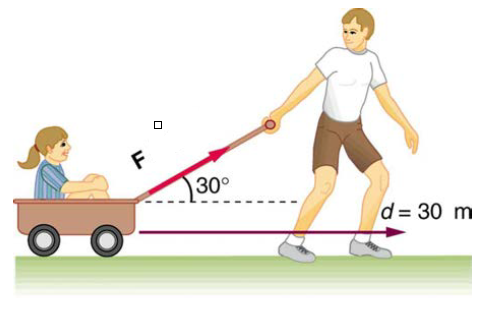# Problem: How much work is done by the boy pulling his sister d = 27.0 m in a wagon as shown in figure below? Assume no friction acts on the wagon and he pulls with a force of 62.0 N and at an angle θ = 31.0°.

###### FREE Expert Solution

Work done:

$\overline{){\mathbf{w}}{\mathbf{=}}{\mathbf{F}}{\mathbf{·}}{\mathbf{d}}{\mathbf{·}}{\mathbf{cos\theta }}}$

Where θ is the angle between the Force and the direction of motion.

90% (327 ratings)###### Problem Details

How much work is done by the boy pulling his sister d = 27.0 m in a wagon as shown in figure below? Assume no friction acts on the wagon and he pulls with a force of 62.0 N and at an angle θ = 31.0°.